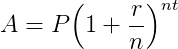Savings Accounts and Annual Percentage Yield

1.   Suppose that you deposit \$500 in a bank that offers an annual percentage rate of 6.0% compounded annually.

a.  What is your account balance after one year?
b.  What is your account balance after 10 years?

2. How long will it take your money to triple at an annual percentage rate of 8% compounded annually?

3.  Suppose that you deposit \$500 in a bank that offers an annual percentage rate of 6.0% compounded monthly.

a.  What is your account balance after one year?
b.  What is your account balance after 10 years?
c.  What is the annual percentage yield for this account? (Recall that the annual percentage yield is the percentage change in the account for one year, in this case in 12 months.)4.  Compare the accumulated balance in three accounts that all start with an initial deposit of \$1000. All three accounts have an annual percentage rate of 5.5%, but the first account compounds interest annually, the second account compounds interest quarterly while the third account compounds interest monthly. Make a table that shows the accumulated balance in all three accounts for the first ten years.

a.  Paste the first ten rows of each table your Word document.
b.  Write a short paragraph discussing the differences between the accounts indicating which account is the largest and which is the smallest.
c.  Calculate the annual percentage yield for each account. Do you want an account that compounds interest annually, quarterly or monthly (given that the annual interest rate is the same)?